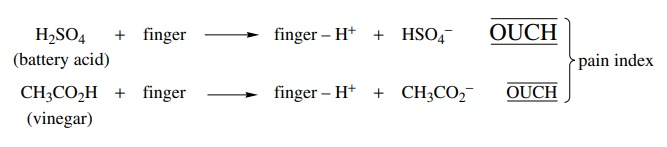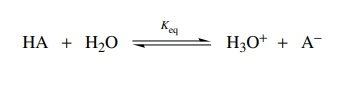# Acid Strength

| Home | | Organic Chemistry |

## Chapter: Organic Chemistry : Acidity and Basicity

Long before we ever took a chemistry course we all had some knowledge of the relative strengths of acids and bases.

ACID STRENGTH

Long before we ever took a chemistry course we all had some knowledge of the relative strengths of acids and bases. If you asked any of your friends if they would rather spill battery acid or vinegar (acetic acid) on their skin, all would choose vinegar. (Even those who have never studied chemistry would make the same choice.) And if you asked them why, they would tell you that battery acid is “stronger” than vinegar. The same people would also prefer to ingest a solution of baking soda (NaHCO3) rather than lye (KOH). Again they know that lye is stronger than soda (although they may not know both are bases). Thus the concept of strong or weak acids and bases is known to more people than you might suspect.

Chemists, however, would rather be a little more specific than describing acids and bases as either strong or weak. They need to be able to put acid or base strength on a quantitative basis. One way to measure the strength of an acid would be to place your index finger into the acid. The ability of an acid to transfer a proton to your index finger, which would act as a base and accept a proton, would result in an audible response—the pain index. From our previous discussion, if battery acid and vinegar were compared by placing the index finger in each, the pain index for battery acid would be much greater than for vinegar because battery acid is “stronger” and can thus transfer a proton to your finger more effectively than vinegar. Other acids could be tested in a similar fashion and their acid strength could be ranked by the audible signal. Since the same base, an index finger, is used to test every acid, the pain index is a direct and quantitative measure of the strength of a given acid compared to the other acids that have been tested. Unfortunately the method is not very reproducible and the sensor can only be used for a limited number of measurements without degradation.But the idea is clear! The strength of an acid can be quantitated by knowing how well it transfers a proton to some standard base. Moreover the extent to which it transfers a proton to the standard base could be compared with the extent to which other acids transfer a proton to the same standard base. Thus, by using a single base, we could not only measure the strength of an acid but also compare acids quantitatively.

To make it simple, let us choose a molecule as the standard base. The acid strength will be a measure of how well the acid transfers a proton to that molecule acting as a base, and we can quantitate the acid strength by measuring the amount of the acid that is ionized in the presence of that base. Thus the ratio of the amount of acid which has transferred a proton to the base compared to the amount of acid which has not transferred a proton to the base is a direct measure of the strength of that acid. Water has been chosen as the standard base molecule (in place of a finger) and the ratio of ionized acid to undissociated acid is called Keq (the equilibrium constant).The equilibrium constant Keq is expressed as the amount of products divided by the amount of reactants present at equilibrium; or(Since two product molecules are produced from two reactant molecules, the ion-ization ratio of the acid is expressed as the product of the product concentrations divided by the product of the reactant concentrations). This numerical ratio can be used to compare the strengths of acids. It is very important to realize just how simple this notion is. The equilibrium constant (in this case the ionization constant of an acid) is merely a ratio of the molecules which have donated a proton to water to those which have not.

To further reduce the complexity, since the concentration of water, [H2O], is a constant in dilute solutions where this treatment is valid, this fraction can be simplified towhich is again essentially a ratio relating the ionized acid molecules to the union-ized acid molecules. For example, if Ka is 1/105, or 105, then one of every 102.5 acid molecules is ionized. On the other hand, if Ka is 106/1, then for every 103 molecules ionized, only one is not ionized. The latter acid is a much stronger acid than the former because a much higher fraction is ionized and donates a proton to water.

We further simplify the comparison by defining pKa = − log Ka because this gives us small numbers to deal with rather than exponentials. It must be noted, however, that pKa units are logarithmic units so one pKa unit represents a change of 1 in 10 in the ratio of acid molecules which are ionized to those which are not. By the use of pKa’s, the larger the number, the weaker the acid. For example, if one compares acetic acid pKa = 4.75 = − log Ka (Ka = 1.8 × 105) with hydrofluoric acid pKa = 3.2 = − log Ka (Ka = 6.3 × 104), it is seen that HF is the stronger acid by about 1.5 orders of magnitude. Likewise, HCl, which has pKa = −6.6 (Ka = 3.98 × 106), is a stronger acid that acetic acid, with pKa = 4.75 (Ka = 1.8 × 105), by more than 10 orders of magnitude (1010 stronger). By using a standard, constant molecule as a base, the measure of the fraction of proton transfer to that base becomes a meaningful and quantitative way to compare acid strengths.Table 3.1 is a compilation of pKa’s of various acids. It is only a representative group of pKa values; literally thousands have been precisely measured. It is evident that, although the acid strengths of organic compounds can vary over 50 orders of magnitude, quantitative comparisons can be made between various acids with good accuracy.